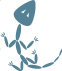Mathematics.Percents (Package)

# Purpose

Percents are commonplace throughout math, and even just as descriptions for things. Having actual percentage types helps correct use of many functions and procedures that need to be constrained as percentages, but would normally rely on integer or float numerics. Percents as a type also allows percentage arithmetic shorthand.

# Percentage Arithmetic

Firstly, it is important to recognize this is only a shorthand for code that would be written out normally. This shorthand not only allows writing it quicker, but also avoids some typing errors.

Every intrinsic one would expect exists, so arithmetic between percents works as expected. This may chance, as something like 12% divided by 6% is unusual and likely nonsensical.

Only Addition and Subtraction with other types are defined, and only with percents on the right hand side. Addition of a percent takes that percent of the left hand value and adds it to the left hand value. As such, `10 + Percent'(50.0)` is 15, because 50% of 10 is 5, and 10 + 5 is 15. Subtraction works the same way, `10 - Percent'(50.0)` is 5, because 50% of 10 is 5, and 10 - 5 is 5.